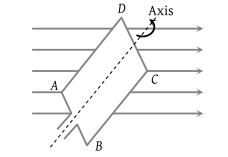• Subject:

...

• Topic:

...

A rectangular coil ABCD is rotated anticlockwise with a uniform angular velocity about the axis shown in diagram below. The axis of rotation of the coil as well as the magnetic field B are horizontal. The induced e.m.f. in the coil would be maximum when(1) The plane of the coil is horizontal

(2) The plane of the coil makes an angle of 45° with the magnetic field

(3) The plane of the coil is at right angles to the magnetic field

(4) The plane of the coil makes an angle of 30° with the magnetic field